# NCERT Miscellaneous Excercise

Question 1: Solve the inequality 2 ≤ 3x – 4 ≤ 5

Answer 1: 2 ≤ 3x – 4 ≤ 5

⇒ 2 + 4 ≤ 3x – 4 + 4 ≤ 5 + 4

⇒ 6 ≤ 3x ≤ 9

⇒ 2 ≤ x ≤ 3

Thus, all the real numbers, x, which are greater than or equal to 2 but less than or equal to 3, are the solutions of the given inequality. The solution set for the given inequality is [2, 3].

Question 2: Solve the inequality 6 ≤ –3(2x – 4) < 12

Answer 2: 6 ≤ – 3(2x – 4) < 12

⇒ 2 ≤ –(2x – 4) < 4

⇒ –2 ≥ 2x – 4 > –4

⇒ 4 – 2 ≥ 2x > 4 – 4

⇒ 2 ≥ 2x > 0

⇒1 ≥ x > 0

Thus, the solution set for the given inequality is (0, 1].

Question 3: Solve the inequality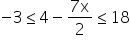Answer 3: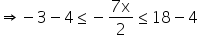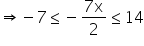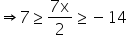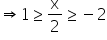⇒2 ≥ x ≥ -4

Thus, the solution set for the given inequality is [–4, 2].

Question 4: Solve the inequality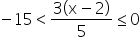Answer 4:⇒ –75 < 3(x – 2) ≤ 0

⇒ –25 < x – 2 ≤ 0

⇒ – 25 + 2 < x ≤ 2

⇒ –23 < x ≤ 2

Thus, the solution set for the given inequality is (–23, 2].

Question 5: Solve the inequality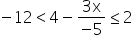Answer 5: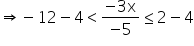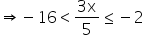⇒ -80 < 3x ≤ -10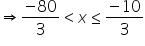Thus, the solution set for the given inequality is.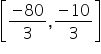Question 6: Solve the inequality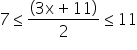Answer 6:⇒ 14 ≤ 3x + 11 ≤ 22

⇒ 14 – 11 ≤ 3x ≤ 22 – 11

⇒ 3 ≤ 3x ≤ 11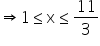Thus, the solution set for the given inequality is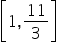Question 7: Solve the inequalities and represent the solution graphically on number line:

5x + 1 > – 24, 5x – 1 < 24

Answer 7: 5x + 1 > –24

⇒ 5x > –25

⇒ x > –5 ……………………………. (1)

5x – 1 < 24 ⇒ 5x < 25

⇒ x < 5 …………………………………. (2)

From (1) and (2), it can be concluded that the solution set for the given system of

inequalities is (–5, 5). The solution of the given system of inequalities can be represented on number line as

Question 8: Solve the inequalities and represent the solution graphically on number line:

2(x – 1) < x + 5,                                    3(x + 2) > 2 – x

Answer 8: 2(x – 1) < x + 5

⇒ 2x – 2 < x + 5

⇒ 2x – x < 5 + 2

⇒ x < 7 …………………………………………… (1)

3(x + 2) > 2 – x ⇒ 3x + 6 > 2 – x ⇒ 3x + x > 2 – 6

⇒ 4x > – 4

⇒ x > – 1 ……………………………………… (2)

From (1) and (2), it can be concluded that the solution set for the given system of inequalities is (–1, 7). The solution of the given system of inequalities can be represented on number line as

Question 9: Solve the following inequalities and represent the solution graphically on number line: 3x – 7 > 2(x – 6), 6 – x > 11 – 2x

Answer 9: 3x – 7 > 2(x – 6)

⇒ 3x – 7 > 2x – 12

⇒ 3x – 2x > – 12 + 7

⇒ x > –5 …………………….… (1)

6 – x > 11 – 2x ⇒ –x + 2x > 11 – 6

⇒ x > 5 ………………………… (2)

From (1) and (2), it can be concluded that the solution set for the given system of inequalities is (5, ∞). The solution of the given system of inequalities can be represented on number line as

Question 10: Solve the inequalities and represent the solution graphically on number line: 5(2x – 7) – 3(2x + 3) ≤ 0, 2x + 19 ≤ 6x + 47

Answer 10: 5(2x – 7) – 3(2x + 3) ≤ 0

⇒ 10x – 35 – 6x – 9 ≤ 0

⇒ 4x – 44 ≤ 0 ⇒ 4x ≤ 44

⇒ x ≤ 11 ………………………………… (1)

2x + 19 ≤ 6x + 47 ⇒ 19 – 47 ≤ 6x – 2x ⇒ –28 ≤ 4x

⇒ –7 ≤ x ……………………………… (2)

From (1) and (2), it can be concluded that the solution set for the given system of inequalities is [–7, 11]. The solution of the given system of inequalities can be represented on number line as

Question 11: A solution is to be kept between 68°F and 77°F. What is the range in temperature in degree Celsius (C) if the Celsius/Fahrenheit (F) conversion formula is given by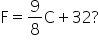Answer 11: Since the solution is to be kept between 68°F and 77°F, 68 < F < 77

Puttingwe obtain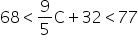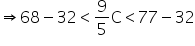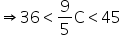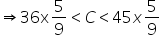⇒ 20 < C < 25

Thus, the required range of temperature in degree Celsius is between 20°C and 25°C.

Question 12: A solution of 8% boric acid is to be diluted by adding a 2% boric acid solution to it. The resulting mixture is to be more than 4% but less than 6% boric acid. If we have 640 litres of the 8% solution, how many litres of the 2% solution will have to be added?

Answer 12: Let x litres of 2% boric acid solution is required to be added.

Then, total mixture = (x + 640) litres

This resulting mixture is to be more than 4% but less than 6% boric acid.

∴ 2%x + 8% of 640 > 4% of (x + 640) and 2% x + 8% of 640 < 6% of (x + 640)

2%x + 8% of 640 > 4% of (x + 640)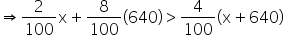⇒ 2x + 5120 > 4x + 2560

⇒ 5120 – 2560 > 4x – 2x

⇒ 5120 – 2560 > 2x

⇒ 2560 > 2x

⇒ 1280 > x

2% x + 8% of 640 < 6% of (x + 640)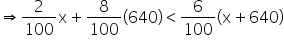⇒ 2x + 5120 < 6x + 3840

⇒ 5120 – 3840 < 6x – 2x

⇒ 1280 < 4x

⇒ 320 < x

∴ 320 < x < 1280

Thus, the number of litres of 2% of boric acid solution that is to be added will have to be more than 320 litres but less than 1280 litres.

Question 13: How many litres of water will have to be added to 1125 litres of the 45% solution of acid so that the resulting mixture will contain more than 25% but less than 30% acid content?

Answer 13: Let x litres of water is required to be added.

Then, total mixture = (x + 1125) litres

It is evident that the amount of acid contained in the resulting mixture is 45% of 1125 litres.

This resulting mixture will contain more than 25% but less than 30% acid content.

∴ 30% of (1125 + x) > 45% of 1125

And, 25% of (1125 + x) < 45% of 1125

30% of (1125 + x) > 45% of 1125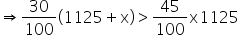⇒ 30(1125+x)>45 x 1125

⇒ 30 x 1125 + 30x > 45 x 1125

⇒ 30x > 45 x 1125 – 30 x 1125

⇒ 30x > (45-30) x 1125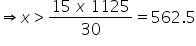25% of (1125 + x) < 45% of 1125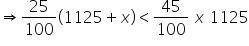⇒ 25(1125+x)>45 x 1125

⇒ 25 x 1125+25x>45 x 1125

⇒ 25x>45 x 1125-25 x 1125

⇒ 25x>(45-25) x 1125

⇒ x >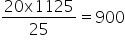∴ 562.5 < x < 900

Thus, the required number of litres of water that is to be added will have to be more than 562.5 but less than 900.

Question 14: IQ of a person is given by the formula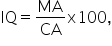Where MA is mental age and CA is chronological age. If 80 ≤ IQ ≤ 140 for a group of 12 years old children, find the range of their mental age.

Answer 14: It is given that for a group of 12 years old children,

80 ≤ IQ ≤ 140 …………………………………. (i)

For a group of 12 years old children, CA = 12 years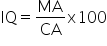Putting this value of IQ in (i), we obtain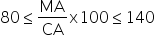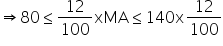⇒ 9.6 ≤ MA ≤ 16.8

Thus, the range of mental age of the group of 12 years old children is 9.6 ≤ MA ≤ 16.8

Related Keywords